# Richer Networks

Tom Kelliher, CS 315

Apr. 19, 1999

### Assignment

Read openings of 3.1, 3.2, 3.3.

### From Last Time

Multiplication, convolution on linear arrays.

### Outline

1. Hypercubes.

2. Butterflies.

### Coming Up

Shuffle-Exchanges.

# The Hypercube

Definition of r-dimensional hypercube:

1. Has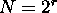nodes and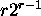edges.

2. Each node is labeled with an r-bit number.

3. Two nodes are connected only if they differ in one bit.

4. Edges partition nicely along dimensions. An edge is called a dimension k edge only if it connects two nodes which differ in the kth bit position.

5. Recursive structure.

Examples: 0- through 4-dimensional hypercubes.

## Properties

1. How can we find a path from node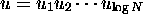to node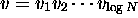?

2. What is the diameter of the graph?

3. What is the bisection width of the graph? (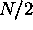--- remove any dimension's edges.)

## Containment of Arrays

1. Mapping a linear array.

1. Hamiltonian cycles.

2. Proof by induction. Basis: N=4.

Step: Partition the N-node hypercube into 2-node hypercubes. Assume that there is an edge (x010, x110) and, symmetrically, (x011, x111) in each sub-hypercube, respectively.

To construct the linear array for the hypercube, remove those edges and add edges (x010, x011) and (x110, x111).

2. Mapping a 2-D array. Example: construct a 4x4 array from a 16 node hypercube.

# The Butterfly

Definition of r-dimensional butterfly:

1. Has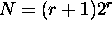nodes and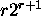edges.

2. Each node is labeled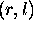, where r (binary) is the row and l (decimal) is the level.

3. Two types of edges:
1. Straight edges connectand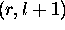.

2. Cross edges connectand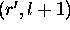, where r and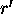differ only in the lth bit position.

3. Recursive structure.

Example: 3-dimensional butterfly.

Observation: Collapsing horizontally, we get the hypercube.

## Properties

1. How can we find a path from nodeto node (r',l)?

Extend to nodes on arbitrary levels.

2. What is the diameter of the graph?

3. What is the bisection width of the graph? Is r correct?

Thomas P. Kelliher
Mon Apr 19 09:48:13 EDT 1999
Tom Kelliher